## Quantification of Atmospheric TEMPERATURE RISE due to Burning of Fossil Fuels on Earth

##### Using (1) Specific Heat Capacity of Air (which is 99% N2+O2) and (2) amount of energy generated each year by burning of fossil fuels (gas, coal, oil) and (3) mass of air in the thin atmospheric shell around Earth.Here is the thin, fragile atmosphere of Earth.
(It is about as thin as the sedimentary layer of the Earth's crust.)

(2019 Nov blog post)

(due to burning of fossil fuels)

! Note !

 INTRODUCTION : In the conversations on 'climate change' and 'global warming', the reporting often talks about 'increased carbon dioxide' (CO2) and 'increased greenhouse gases' (GHG) rather than the more directly experienced ('less removed') factors 'increased heat generation' and 'accelerating temperature rise'. I have pointed out, on another page of this web site, on climate change, atmospheric gases, and fossil fuel heat generation that the modeling of the SOURCES AND CAUSES OF ATMOSPHERIC TEMPERATURE RISE ARE VERY COMPLEX AND INVOLVE MUCH MORE THAN infrared radiation and capture of that radiation by carbon dioxide --- which is consitutes only 4 HUNDREDTHS OF ONE PERCENT of Earth's atmosphere. In short, there are other factors (other than carbon dioxide) that may be contributing significantly to atmospheric temperature rise. Nitrogen molecules (N2) and oxygen molecules (O2) constitute 99 PERCENT OF THE ATMOSPHERE (about 78% N2 and 21% O2). The 'science', that is often referred to, was an isolated experiment in a laboratory setting (in the mid-1800's by an English physicist, John Tyndall) --- comparing infrared radiation absorption by various gases --- such as CO2 and N2 and O2. He showed that a flask of CO2 absorbs much more infrared radiation than N2 or O2. However, note that because N2 and O2 make up about 99% of the atmosphere and CO2 makes up about 0.04%, there is about 99/0.04 = 2,475 times more N2 and O2 in the atmosphere than CO2. Statements about causes of atmospheric temperature rise typically ignore the infrared absorption of N2 and O2. That implies that the people making these statements believe that N2 and O2 absorb on the order of 1% (or less) as much radiation as CO2. Put that factor of 100 difference together with the fact that N2 plus O2 are more than 2,475 times as plentiful as CO2, and we find that such statements (and the people who make those statements) imply that the infrared absorption of N2 and O2 should be about 247,500 times less than CO2. Is that the case? Does CO2 dominate (by that much) N2 and O2 in the absorption of infrared radiation? I have done a lot of web searches for documents that might compare the infrared absorption of CO2 to N2 and O2. No luck. The absorption data for N2 and O2 seems to be very hard to find. Perhaps you will have better luck with WEB SEARCHES on keywords such as I suspect that infrared radiation absorption by N2 and O2 is not that 'negligible'. If the infrared absorption by N2 and O2 is not negligible, then there is EVEN MORE trapping of energy by atmospheric gases. But let us put that infrared radiation absorption issue aside for now --- and proceed to consider another possible source of atmospheric temperature rise --- 'direct heating' of N2 and O2 molecules. That is, let us consider the increase in the average velocities of the N2 and O2 molecules due to 'direct heating' from heat sources here on Earth. The point of this page I think that there is too much talk about the causes of 'climate change' --- that is, 'atmospheric temperature rise' --- WITHOUT any consideration of the effect that the burning of fossil fuels (gas, coal, oil) has 'directly' on the atmosphere. In other words, the conversation seems to be focused only on the amount of heat in the atmosphere due to 'trapped' solar radiation --- with no consideration of the 'direct effect' of fossil fuel burning on the temperature of the atmosphere. By 'direct effect', I mean the heating of the N2 and O2 (and other) molecules in 'local' air (initially) by direct contact with 'heated items' that result from fossil fuel burning. These 'heated items' can include hot gases from the burning of gas-coal-oil-biomass hot surfaces such as the engine blocks, manifolds, and exahust pipes of combustion engines heat from ovens and furnaces, used for baking, paint curing, metal extraction and metal melting, ceramic hardening, etc. heat from the heating systems of residential and commercial buildings and the list could go on. 'Initially' that heated atmosphere is in the locality of the heat source, but those heated N2 and O2 molecules rapidly share their increased kinetic energy (molecule speed-ups) with more remote atmospheric molecules by direct collisions near the localities and by being carried afar by wind currents (both horizontal and vertical), and then 'sharing' their increased kinetic energy with 'remote' atmospheric molecules. I think we can all agree that this 'equilibration' of the molecular velocities will occur within a matter of days --- certainly within a few weeks. In this web page, I WANT TO PRESENT A CALCULATION OF THE ATMOSPHERIC TEMPERATURE RISE (per year) DUE TO THE BURNING OF FOSSIL FUELS (at the present rate). This calculation will involve three basic factors: the specific heat capacity of air the amount of energy produced/consumed (per year) by humans by burning fossil fuels on Mother Earth the mass of air in the Earth's atmospheric shell. This calculation will yield a rough, 'ball park' estimate. Let us consider those 3 factors in more detail. (You can perhaps make improvements in the calculation of each factor.) The first factor --- specific heat of air --- is known sufficiently accurately. We will use 1,012 joules per kilogram per degree Centigrade. (This figure was taken from the 'isobaric mass heat capacity' column of a Table of specific heat capacities.) Note that 'specific heat' is the amount of energy that must be added, in the form of heat, to one unit of mass of the substance (air in this case) in order to cause an increase of one unit in its temperature. So 'specific heat' involves three units of measure --- for heat (energy), mass, and temperature. And 'specific heat' is heat-added, divided by a unit-of-mass, further divided by a unit of temperature. Typical units of measure are joules for heat energy kilograms for mass degree-Kelvin (or, equivalently, degree-Centigrade) for temperature So a value for 'specific heat' of a material is typically joules per kilogram per degree-Centigrade --- which can be more briefly written as joules/kg-degC. The second factor above --- annual fossil fuel heat generation or consumption by humans --- is known pretty accurately. It has been reported in many financial publications over the past several decades. It has been reported for individual fossil fuels (gas, oil, coal) --- AND as a total. The energy per year (in joules per year) generated from fossil fuel sources is about 5.6 x 10^20 joules/year (around 2015) as reported in an article titled 'How much fuel does it take to power the world?' by Ethan Siegal for Forbes magazine. If you want to put the total-joules together by getting data for gas and coal and oil separately, and then adding them up, you could try WEB SEARCHES on keywords like: to accumulate the necessary data. If you find energy data in other energy units (such as BTU's, calories, kilowatt-hours, or other), you could convert to joules. The third factor above --- the number of kilograms of air affected by all this fossil fuel burning --- is a little more complicated to calculate. But it definitely has an upper bound as can be seen in the image at the top of this page, that shows the atmosphere of Earth is a thin shell of gas (most of it within 5 miles of the surface of the Earth). The height of a significant 'majority' of this gas is indicated by the fact that if you hike to the top of the highest mountains (about 5 miles high), you will need an oxygen mask. Given that the radius of the Earth is about 4,000 miles, we see that the height of that shell of atmospheric gas is only 5/4000 = 0.125 percent of the radius of the Earth --- about one-tenth of 1 percent. This is a very thin shell as indicated by the thin black line (a circle arc) in the following cross-sectional diagram of Earth --- where the thin line represents Earth's atmospheric shell.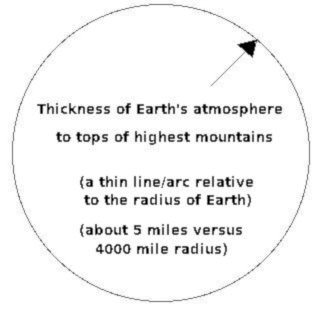If you put this circle in a screen area of 1000x1000 pixels, the 8,000 mile diameter of the circle would imply that each pixel represents 8 miles. So that 5-mile thick shell of air would be represented by less than a pixel at each point around the circle. By using the formula for the volume of a sphere, we can calculate the volume of that thin shell of atmosphere --- say in cubic kilometers. Then by using the average density of the air in that shell (say, in kilograms per cubic meter), we can calculate the mass of that atmospheric shell (in kilograms) --- by using a couple of multiplications. The calculation of the mass of the atmosphere (in kilograms) will be presented in an appendix-section below. Suffice it to say that the Earth's atmospheric shell contains about 5.10 x 10^18 kilograms of air where the symbol '^' represents exponentiation. Putting the 3 factors together We can use the 3 factors above to arrive at the expected atmospheric temperature rise per year --- in Centigrade per year --- by multiplying the three factors together, as follows. Let Sair = the Specific heat of air. Let Mair = the Mass of the shell of air. Let FFEpY = the Energy generated per Year by burning Fossil Fuels Let ATRpY = the Atmospheric Temperature Rise per Year Then we will use the simple division-and-multiplication formula ATRpY = (1 / Sair) x (1 / Mair) x FFEpY We can check that the right side gives us the proper units of measure for the result by noting that the right side units are (kg x degC / joules) x (1 / kg) x joules/year The 'kilograms' and the 'joules' cancel out, leaving us with degC / year. The arithmetic Here is a presentation of the arithmetic. ATRpY = (1 / 1,012) x (1 / 5.10 x 10^18) x 5.60 x 10^20 The 10-to-the-twentieth divided by 10-to-the-eighteenth results in a factor of 100. And 1,012 x 5.10 = 5161.2 So ATRpY = (5.60 / 5161.2) x 100 = 0.1085 degCentigrade/year Because of the 'roughness' of these factors, we should keep only the first couple of 'significant' digits. So we estimate the 'rate of increase of the atmospheric temperature per year --- due to burning of fossil fuels' to be 0.10 of a degree-Centigrade per year --- which is almost 0.2 degrees Fahrenheit per year. So in 10 years (~2030), we can expect almost 1.0 degree-Centigrade atmospheric temperature rise --- which is almost 2 degrees Fahrenheit. In 20 years (~2040), we can expect about 2.0 degC temperature rise --- due to burning of fossil fuels --- ON TOP OF any atmospheric temperature rise due to 'greenhouse gases'. In 40 years (~2060), we can expect about 4.0 degC temperature rise --- due to burning of fossil fuels. Note that these are overestimates because a large amount of heat energy in the air is transferred into ice, ocean water, and land masses. (Taking those factors into account would take more complex mathematical climate models, as discussed below.) --- The following plot of global temperature change indicates that the temperature at the surface of planet Earth has been on an upward trend since (at least) about 1975.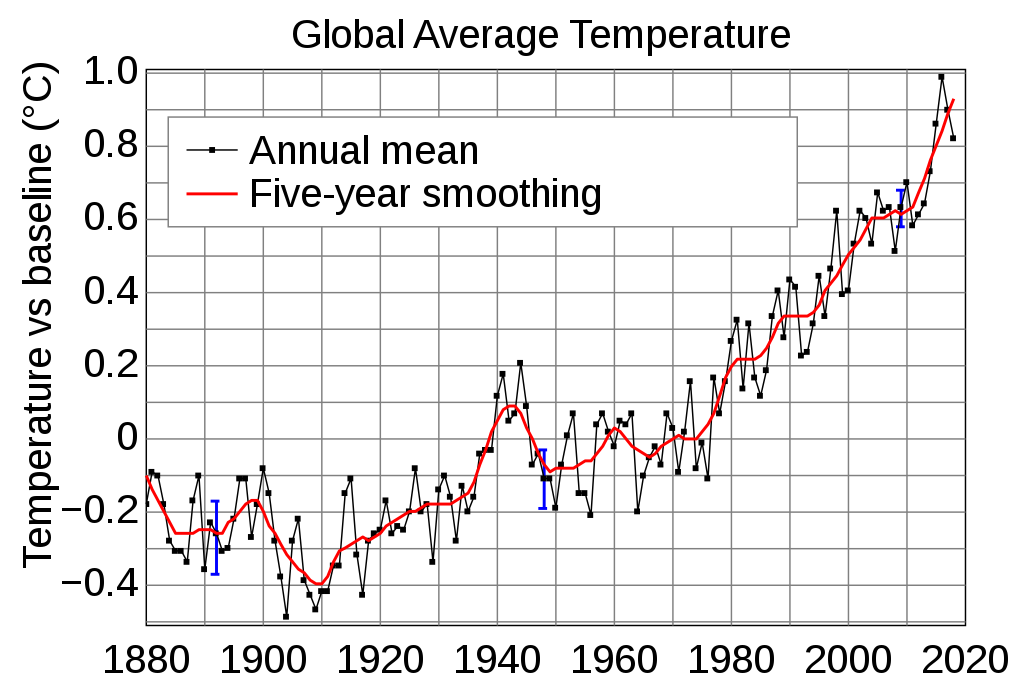from Wikipedia page named Instrumental Temperature Record This graph (if it represents AIR temperature at the surface of Earth) indicates that the rate of change is about 0.9 deg-Centigrade over a period of about 40 years (1975-2015) --- which is an increase of about 0.9 / 40 = 0.0225 deg-C per year. This is in rough agreement with what one would expect from the 'direct heating' of air by burning of fossil fuels (about 0.1 degC/yr) --- in addition to the 'trapping' of solar infrared radiation by greenhouse gases ... especially if one considers that ... when the cooling effect of Arctic-Greenland-Antarctic-glacier ice is terminated when that ice melts away, we may see the rate-of-increase of planet temperatures increase significantly from about 0.02 deg-C per year. Also, consider that some of the heat in the air is transferred into the oceans --- and into the land masses --- thus lowering the temperature rise of the air. That 'bump' around 1939-1945 is probably due to the increase in heat from accelerated manufacturing and consumption of fuel by planes, ships, tanks, and other vehicles during World War II. The following plot of global temperature change, over more than a thousand years, indicates that the temperature at the surface of planet Earth has gone 'on a tear' from about 1850 to 2015 --- in other words, ever since the 'Industrial Revolution' --- ever since burning of coal, and then oil-and-gas, increased drastically --- helped along by a recent explosion in human population on the planet (see population graphs below).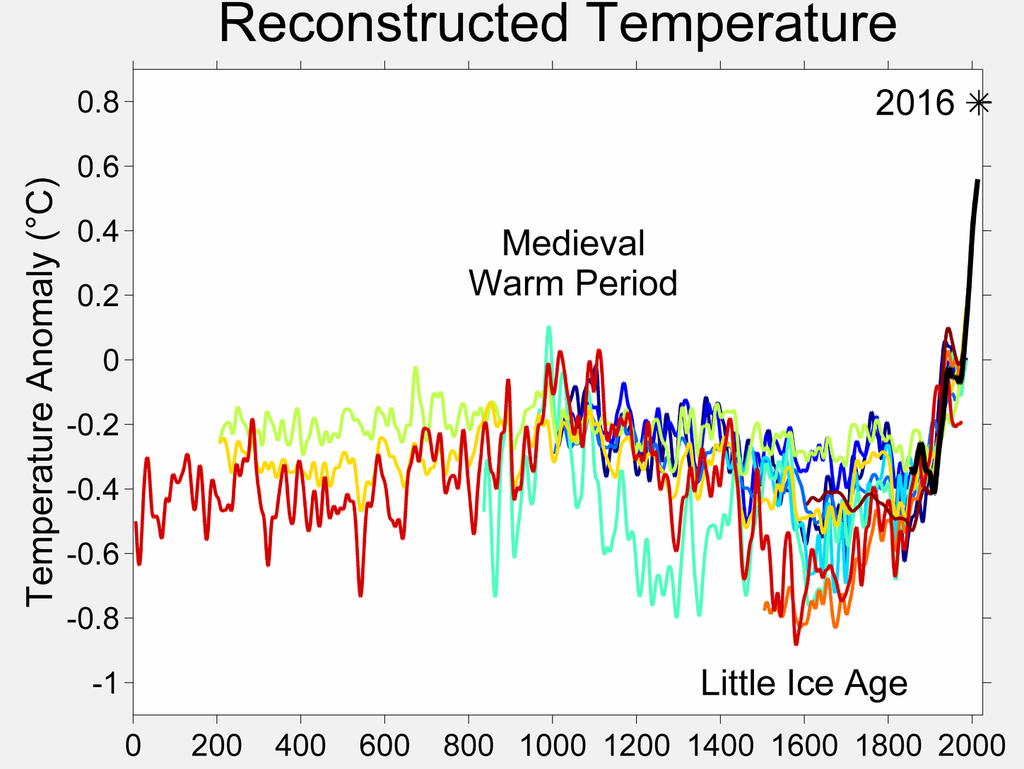from Wikipedia page named Temperature Record of the past 1000 years You could do a WEB SEARCH on keywords such as to find other (and more current) data on atmospheric temperature rise. Note that, because the larger cities of the world probably have a good temperature record since about 1850 or 1900, you could build your own temperature graphs (over a period of about 1900 to the present) using data from cities scattered over the Northern and Southern Hemispheres --- cities such as New York City, New York San Francisco, California Houston, Texas Chicago, Illinois Toronto, Canada Mexico City, Mexico Rio de Janiero, Brazil Buenos Aires, Argentina Santiago, Chile Lima, Peru London, England Paris, France Madrid, Spain Vienna, Austria Istanbul, Turkey Cairo, Egypt Nairobi, Kenya Capetown, South Africa Lagos, Nigeria Moscow, Russia Shanghai, China Tokyo, Japan Melbourne, Australia etc. A plot of the average of all the city temperatures (using, say, average temperatures in each month of the year) --- or a plot of the individual city temperature curves on one graph --- over the period 1900 to present --- would, no doubt, confirm the shape of the above graphs. --- These air-temperature-rise figures (about 0.02 to 0.1 deg-C per year) do not sound like much of a temperature rise, but, since this applies all over the globe, it will definitely result in turning the Middle East (and Equatorial regions) into unbearable hot boxes --- which is already happening in 2019 turning low-lying islands in the Pacific and Indian Ocean (and elsewhere) into submerged reefs --- which is already happening in 2019 turning massive snow and ice fields in mountains, in the Arctic, in Greenland, and in the Antarctic into ice-less regions (which will leave Earth even more subject to accelerated air temperature increases when the buffering effect of the heat of melting of ice is no longer available) --- which is already happening in 2019 turning the state of California into an annual forest fire tinderbox, with loss of thousands of homes and generation of an atmosphere of choking, unhealthy smoke --- which is already happening in 2019 and this list could go on and on and on and ... especially as the generation of 'old-solar-energy' by burning unearthed fossil-fuels is COUPLED WITH accumulation of 'current-solar-energy' in the form of infrared radiation being 'trapped' by greenhouse gases. --- The effects on Mother Earth may be coming faster than this analysis suggests. For example, the human population growth on Earth is exploding --- as graphs like the following show.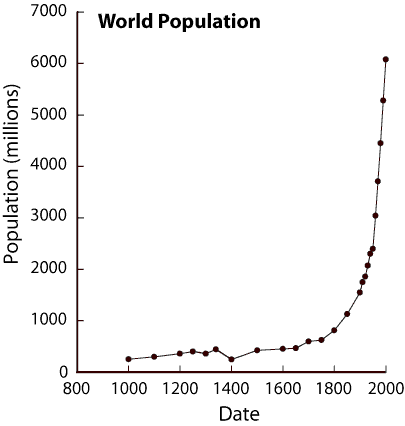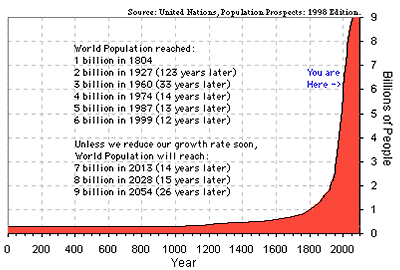Just as the human population is expected to increase about 50% (about 4 billion people) in the time period 2020-2040, the burning of fossil fuels can be expected to increase about 50% as well (by about 3 x 10^20 additional joules per year) --- if those fuels last that long and are affordable that long. So the temperature-rise-per-year estimates above are under-estimates, because fossil-fuel use will probably increase significantly in coming years --- unless humans come to their senses and use non-fossil-fuel (and non-radioactive, non-extremely-dirty) energy sources such as wind turbines, solar-panels, hydro-electric dams, ocean energy, and geothermal energy sources --- in place of the fossil fuels (and long-lived radioactivity) being used for electricity generation. The fossil fuels could continue to be used for many years for purposes such as truck, airplane, train, and ship propulsion. But there could be a gradual migration to other propulsion methods --- such as electric trains instead of diesel trains, and electric buses instead of diesel buses. Complicated heat transfer issues: There are complicating factors for computations like these, such as the kinetic energy in all those N2 and O2 molecules will transfer some of their energy into the ice and snow on Earth's oceans and land masses --- in which the heat of melting represents a huge energy 'sink' the kinetic energy in all those N2 and O2 molecules will transfer some of their energy into the watery/fluid/flowing surface of the Earth's oceans the kinetic energy in all those N2 and O2 molecules will transfer some of their energy into the solid surface of the Earth's land masses. I welcome anyone to build computer models of the complex heat transfer processes going on every day on Mother Earth. Like the computer models of 'near-term' weather changes, there can be many different 'long-term temperature rise' computer models --- each giving somewhat different (or drastically different) results. Here 'near-term' means about a week, and 'long-term' means about 5 decades. The results of 'near-term' climate models provide the graphic predictions that we see on TV station weather reports every day. The 'long-term' models that consider only 'greenhouse gas' effects WITHOUT considering the 'direct heating' effect of fossil fuels can be expected to give 'drastically different' results from those models that do consider 'direct heating'. I leave it to others to devise such complex models --- perhaps to be run on supercomputers (which I do not have) --- perhaps to simulate the evolution of heat flows both in time and at specific 3D-coordinates near the surface of the Earth --- using numerical-integration of complex systems of differential equations. Note that the developers of 'long term' climate models could use the world temperature data from about 1850 to 2000 (as seen in graphs above) to help validate their models to a certain extent. --- I 'bail out' here with the following observation. If 'near-term' weather models (used daily in weather reports on TV news channels) are found someday to give much better predictive results by taking into account the heat being generated by urban and manufacturing areas of the planet, then that will give some credence to my thesis here : Namely, that the 'direct heating' of N2 and O2 molecules (by the burning of fossil fuels) has a significant effect on the rising temperature of Earth's atmosphere --- perhaps an even greater effect than the effect of solar radiation trapped by 'greenhouse gases'. The fossil fuel companies --- like Exxon-Mobil and coal companies --- have probably known this for years, but do not want the general populace to know this. The fossil fuel companies probably prefer that people think CO2 is the only culprit, so that they can get big government contracts to build CO2 'sequestration' boondoggle projects. (The oil companies use that 'sequestered' CO2 gas pressure to get even more fossil fuel out of the ground. Someday these boondoggles will be exposed, it is just a matter of time.) In Conclusion: Ironically, the Middle East (a major source of fossil fuel) is complicit in turning its region of the world into an unbearable hot box that is quite unfriendly to human life. Good luck, future generations of humans (and other life) on Earth.

 APPENDIX - Calculation of Mass of Air in a thin shell around Earth: We can use 2 factors to arrive at the mass of the shell of air around the Earth --- (1) volume of the spherical shell and (2) average density of the air. Let Vair = the Volume of the spherical shell of air. Let Dair = the average Density of the air in that shell. Let Mair = the Mass of air in that shell. Then we will use the simple multiplication formula Mair = Vair x Dair where Dair is about 1.225 kg/m^3 at sea level. Computation of Vair: First we compute Vair by using the formula Vair = 4/3 x pi x (R^3 - r^3) where r is the radius of Earth (about 6336 kilometers) and R is the radius of Earth plus the height of the major part of the atmosphere (about 6371 kilometers). Then R^3 = 259,816,204,341 km^3 r^3 = 258,596,602,811 km^3 R^3 - r^3 = 1,219,601,530 km^3 4/3 x pi = 4.18877 So Vair = 4/3 x pi x (R^3 - r^3) = 4.18877 x (1,219,601,530 km^3) x (10^9 m^3/km^3) = 4.1887 x 1.2196 x (10^18 m^3) = 5.1085 x 10^18 m^3 Computation of Dair: As mentioned above, the density of air at sea level is about 1.225 kg/m^3. At a height of about 4 to 5 miles above sea level, the density of air will be about half that figure --- about 0.6 kg/m^3. We could expect the AVERAGE density of air in the 5-mile thick shell around Earth to be between 0.6 and 1.225 kg/m^3 --- about 0.9 kg/m^3. But let us boost that 'average-density-of-air' to 1.0 kg/m^3 --- to take into account that there is a small amount of air above the 5-mile height --- and that relatively small mass of air could be taking part in the 'equilibration' of N2 and O2 molecule velocities. Computation of Mair: So, using the above estimates of Vair and Dair, we get Mair = Vair x Dair = (5.1085 x 10^18 m^3) x 1.0 kg/m^3 = 5.10 x 10^18 kg If you think that this is an inaccurate value for the mass of the shell of air around the Earth, you are right. It is a 'ball-park' estimate. Admittedly, this is a very rough way of dealing with the volume and density of air in the atmospheric shell around Earth, but we will show below that this is not an unreasonable value for 'Mair', the mass of air around the Earth. If you think you have a better method and estimate, you can use your estimate to plug into the computation above that provides an estimate of the yearly atmospheric temperature rise due to burning of fossil fuels. The density of the atmosphere falls off in an approximately exponential fashion which can be estimated by the Barometric formula (Wikipedia link). So one could estimate the mass of the air by doing some spherical numerical-integration of an air-density function (or table of data) which varies with the distance above the surface of the Earth. The numerical-integration could be done by summing up the mass of 'differential shells' of the atmosphere above the surface of the Earth. In fact, somebody probably did a numerical-integration like this to get a value for the 'total mean mass of the atmosphere' of 5.1480 × 10^18 kg (Wikipedia link), which is very close to the 5.10 × 10^18 kg value we arrived at above using 'rough reasoning'. --- There could be some 'complicating factors' that would cause some modification to the value of 'Mair' and the other factors in the computations above. For example ... The question of how to handle the transfer of energy from relatively high-density portions of the LOWER atmosphere to very low-density portions of the atmosphere at HIGH altitudes brings in further complications. (Is there much transfer? Should we ignore low-density air at very high altitudes?) Also ... There may be significant effects on these 'ball park' calculations if we were to take into account water vapor in the air --- and methane gas leaks and venting into the air. Consideration of TEMPERATURE effects on density at various levels of the atmosphere --- for example, low temperatures at high altitudes --- can result in more headaches in devising an appropriate computation method. In any case, I expect that the relatively simple arithmetic calculations presented above yield a good 'ball park' estimate of the atmospheric temperature rise per year due to burning of fossil fuels.

Bottom of this blog page on
Quantification of Temperature Rise
of Earth's Atmosphere due to
Burning of Fossil Fuels
.

OR, ...

##### < Go to Top of Page, above. >

Page history:

Page was posted 2019 Nov 11.

Page was changed 2019 Nov 12.